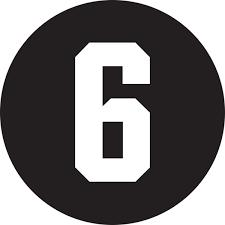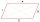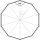How many square meters is needed to cover the tower the shape of regular quadrangular pyramid base edge 10 meters, if the deviation lateral edges from the base plane is 68 °? Calculate coverage waste 10%.

Correct result:

S =  400.438 m2

#### Solution:

$h = (a/2)\cdot \sqrt2 \cdot \tan 68 ^\circ = 17.502 \ \\ h_2 = \sqrt{ h^2 + (a/2)^2 } = 18.202 \ \\ S_1 = a h_2 / 2 = 91.01 \ \\ S = 4 \cdot S_1 \cdot 1.1 = 400.438 \ \text{m}^2$We would be very happy if you find an error in the example, spelling mistakes, or inaccuracies, and please send it to us. We thank you!Tips to related online calculators
Pythagorean theorem is the base for the right triangle calculator.

#### You need to know the following knowledge to solve this word math problem:

We encourage you to watch this tutorial video on this math problem:

## Next similar math problems:The regular quadrilateral pyramid has a base edge a = 1.56 dm and a height h = 2.05 dm. Calculate: a) the deviation angle of the sidewall plane from the base plane b) deviation angle of the side edge from the plane of the base
• Distance of pointsA regular quadrilateral pyramid ABCDV is given, in which edge AB = a = 4 cm and height v = 8 cm. Let S be the center of the CV. Find the distance of points A and S.In a regular quadrilateral pyramid, the height is 6.5 cm and the angle between the base and the side wall is 42°. Calculate the surface area and volume of the body. Round calculations to 1 decimal place.The height of a regular quadrilateral prism is v = 10 cm, the deviation of the body diagonal from the base is 60°. Determine the length of the base edges, the surface, and the volume of the prism.
• Find theFind the content of a regular 12 sided polygon, if its side a = 12 cm.
• WhichWhich of the following numbers most accurately area of a regular decagon with side s = 2 cm? (A) 9.51 cm2 (B) 20 cm2 (C) 30.78 cm2 (D) 31.84 cm2 (E) 32.90 cm2
• SailboatThe 20 m long sailboat has an 8 m high mast in the middle of the deck. The top of the mast is fixed to the bow and stern with a steel cable. Determine how much cable is needed to secure the mast and what angle the cable will make with the ship's deck.
• An observerAn observer standing west of the tower sees its top at an altitude angle of 45 degrees. After moving 50 meters to the south, he sees its top at an altitude angle of 30 degrees. How tall is the tower?
• The towerThe observer sees the base of the tower 96 meters high at a depth of 30 degrees and 10 minutes and the top of the tower at a depth of 20 degrees and 50 minutes. How high is the observer above the horizontal plane on which the tower stands?
• Powerplant chimneyFrom the window of the building at a height of 7.5 m, the top of the factory chimney can be seen at an altitude angle of 76° 30 ′. The base of the chimney can be seen from the same place at a depth angle of 5° 50 ′. How tall is the chimney?
• Traffic signThere is a traffic sign for climbing on the road with an angle of 7%. Calculate at what angle the road rises (falls).
• FighterA military fighter flies at an altitude of 10 km. From the ground position, it was aimed at an altitude angle of 23° and 12 seconds later at an altitude angle of 27°. Calculate the speed of the fighter in km/h.
• TV towerCalculate the height of the television tower if an observer standing 430 m from the base of the tower sees the peak at an altitude angle of 23°?The ladder touch on a wall at a height of 7.5 m. The angle of the inclination of the ladder is 76°. How far is the lower end of the ladder from the wall?From the observation tower at a height of 105 m above sea level, the ship is aimed at a depth angle of 1° 49´. How far is the ship from the base of the tower?The bases of the isosceles trapezoid ABCD have lengths of 10 cm and 6 cm. Its arms form an angle α = 50˚ with a longer base. Calculate the circumference and content of the ABCD trapezoid.The rescue helicopter is above the landing site at a height of 180m. The site of the rescue operation can be seen from here at a depth angle of 52° 40 '. How far will the helicopter land from the rescue site?